# Ohm’s Law

## Basic Electricity

• #### Question 1

For a given amount of water pressure, which will flow a greater rate of water: a small (restrictive) nozzle or a large (unrestrictive) nozzle? Explain how this relates to the study of voltage, current, and resistance in a simple electric circuit.• #### Question 2

Suppose you were to build this circuit and take measurements of current through the resistor and voltage across the resistor:Recording these numerical values in a table, the results look something like this:

 XXXXXXX XXXXXXX

 Current Voltage

 0.22 A 0.66 V

 0.47 A 1.42 V

 0.85 A 2.54 V

 1.05 A 3.16 V

 1.50 A 4.51 V

 1.80 A 5.41 V

 2.00 A 5.99 V

 2.51 A 7.49 V

Plot these figures on the following graph: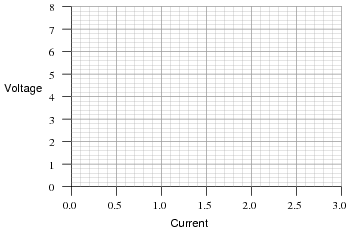What mathematical relationship do you see between voltage and current in this simple circuit?

• #### Question 3

Explain, step by step, how to calculate the amount of current (I) that will go through the resistor in this circuit: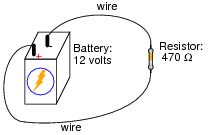• #### Question 4

Plot the relationships between voltage and current for resistors of three different values (1 Ω, 2 Ω, and 3 Ω), all on the same graph: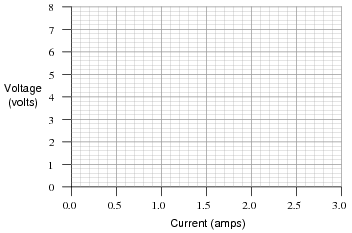What pattern do you see represented by your three plots? What relationship is there between the amount of resistance and the nature of the voltage/current function as it appears on the graph?

Advanced question: in calculus, the instantaneous rate-of-change of an (x,y) function is expressed through the use of the derivative notation: $$\frac{dy}{dx}$$. How would the derivative for each of these three plots be properly expressed using calculus notation? Explain how the derivatives of these functions relate to real electrical quantities.

• #### Question 5

What is the value of this resistor, in ohms (Ω)?• #### Question 6

A common saying about electricity is that ït always takes the path of least resistance.” Explain how this proverb relates to the following circuit, where electric current from the battery encounters two alternate paths, one being less resistive than the other:• #### Question 7

One style of light bulb, very different from the ïncandescent” design which works on the principle of a super-heated wire filament emitting light, is called a gas discharge tube. In this design of light bulb, light is produced by the direct ëxcitation” of gas molecules as electric current passes between two electrodes:Both types of light bulbs have interesting voltage/current plots, neither one being identical to the voltage/current plot of a resistor. First, the voltage/current plot for an incandescent light bulb: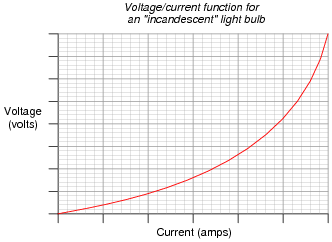Next, the voltage/current plot for a gas-discharge light bulb: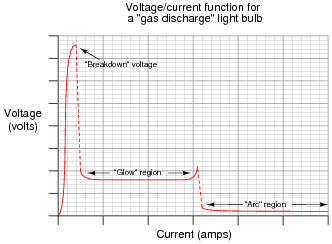Based on these two graphs, what can you say about the electrical resistance of each bulb type over its operating range?

• #### Question 8

Draw the schematic diagram for an experimental circuit to gather data necessary to plot the voltage/current graph of a gas discharge lamp.

• #### Question 9

What is negative resistance?

• #### Question 10

What would happen if a wire having no resistance at all (0 Ω) were connected directly across the terminals of a 6-volt battery? How much current would result, according to Ohm’s Law?Suppose we were to short-circuit a 6-volt battery in the manner just described and measure 8 amps of current. Why don’t the calculated figures from the previous paragraph agree with the actual measurement?

• #### Question 11

Shunt resistors are often used as current-measuring devices, in that they are designed to drop very precise amounts of voltage as large electric currents pass through them. By measuring the amount of voltage dropped by a shunt resistor, you will be able to determine the amount of current going through it: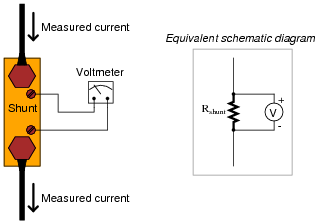Suppose that a shunt resistance is labeled with the following rating: 150 A , 50 mV. What is the resistance of this shunt, in ohms? Express your answer in metric notation, scientific notation, and plain decimal notation.

• #### Question 12

 Don’t just sit there! Build something!!

Learning to mathematically analyze circuits requires much study and practice. Typically, students practice by working through lots of sample problems and checking their answers against those provided by the textbook or the instructor. While this is good, there is a much better way.

You will learn much more by actually building and analyzing real circuits, letting your test equipment provide the “answers” instead of a book or another person. For successful circuit-building exercises, follow these steps:

1. Carefully measure and record all component values prior to circuit construction.
2. Draw the schematic diagram for the circuit to be analyzed.
3. Carefully build this circuit on a breadboard or other convenient medium.
4. Check the accuracy of the circuit’s construction, following each wire to each connection point, and verifying these elements one-by-one on the diagram.
5. Mathematically analyze the circuit, solving for all values of voltage, current, etc.
6. Carefully measure those quantities, to verify the accuracy of your analysis.
7. If there are any substantial errors (greater than a few percent), carefully check your circuit’s construction against the diagram, then carefully re-calculate the values and re-measure.

Avoid very high and very low resistor values, to avoid measurement errors caused by meter “loading”. I recommend resistors between 1 kΩ and 100 kΩ, unless, of course, the purpose of the circuit is to illustrate the effects of meter loading!

One way you can save time and reduce the possibility of error is to begin with a very simple circuit and incrementally add components to increase its complexity after each analysis, rather than building a whole new circuit for each practice problem. Another time-saving technique is to re-use the same components in a variety of different circuit configurations. This way, you won’t have to measure any component’s value more than once.

• #### Question 13

One of the fundamental equations used in electricity and electronics is Ohm’s Law: the relationship between voltage (E or V, measured in units of volts), current (I, measured in units of amperes), and resistance (R, measured in units of ohms):

$$E=IR \ \ \ \ \ \ \ \ \ \ \ I=\frac{E}{R} \ \ \ \ \ \ \ \ \ \ \ R=\frac{E}{I}$$

Where,

E = Voltage in units of volts (V)

I = Current in units of amps (A)

R = Resistance in units of ohms (Ω)

Solve for the unknown quantity (E, I, or R) given the other two, and express your answer in both scientific and metric notations:

I = 20 mA, R = 5 kΩ; E =

I = 150 μA, R = 47 kΩ; E =

E = 24 V, R = 3.3 MΩ; I =

E = 7.2 kV, R = 900 Ω; I =

E = 1.02 mV, I = 40 μA; R =

E = 3.5 GV, I = 0.76 kA; R =

I = 0.00035 A, R = 5350 Ω; E =

I = 1,710,000 A, R = 0.002 Ω; E =

E = 477 V, R = 0.00500 Ω; I =

E = 0.02 V, R = 992,000 Ω; I =

E = 150,000 V, I = 233 A; R =

E = 0.0000084 V, I = 0.011 A; R =

• #### Question 14

A quantity often useful in electric circuit analysis is conductance, defined as the reciprocal of resistance:

$$G= \frac{1}{R}$$

The unit of conductance is the siemens, symbolized by the capital letter “S”. Convert the following resistance values into conductance values, expressing your answers in both scientific and metric notations:

R = 5 k Ω ; G =

R = 47 Ω ; G =

R = 500 M Ω ; G =

R = 18.2 μΩ ; G =

Now, algebraically manipulate the given equation to solve for R in terms of G, then use this new equation to work “backward” through the above calculations to see if you arrive at the original values of R starting with your previously calculated values of G.

• #### Question 15

Suppose an electric current of 1.5 microamps (1.5 μA) were to go through a resistance of 2.3 mega-ohms (2.3 MΩ). How much voltage would be “dropped” across this resistance? Show your work in calculating the answer.

• #### Question 16

One of the fundamental equations used in electricity and electronics is Ohm’s Law: the relationship between voltage (E or V, measured in units of volts), current (I, measured in units of amperes), and resistance (R, measured in units of ohms):

$$E=IR \ \ \ \ \ \ \ \ \ \ \ I=\frac{E}{R} \ \ \ \ \ \ \ \ \ \ \ R=\frac{E}{I}$$

Where,

E = Voltage in units of volts (V)

I = Current in units of amps (A)

R = Resistance in units of ohms (Ω)

Solve for the unknown quantity (E, I, or R) given the other two, and express your answer in both scientific and metric notations:

I = 45 mA, R = 3.0 kΩ; E =

I = 10 kA, R = 0.5 mΩ; E =

E = 45 V, R = 4.7 kΩ; I =

E = 13.8 kV, R = 8.1 kΩ; I =

E = 500 μV, I = 36 nA; R =

E = 14 V, I = 110 A; R =

I = 0.001 A, R = 922 Ω; E =

I = 825 A, R = 15.0 mΩ; E =

E = 1.2 kV, R = 30 MΩ; I =

E = 750 mV, R = 86 Ω; I =

E = 30.0 V, I = 0.0025 A; R =

E = 0.00071 V, I = 3389 A; R =

### Related Content

Published under the terms and conditions of the Creative Commons Attribution License